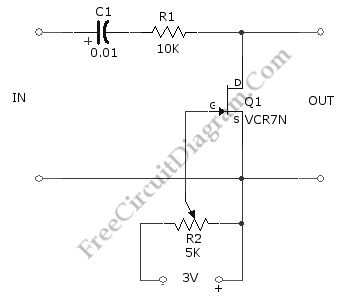# Voltage-Controlled Attenuator (Volume Control) Using FETUsing the circuit shown in the schematic diagram below, we can control the low-level audio signals with ±3V variable DC voltage. This attenuator circuit uses a field effect transistor (FET) to shunt the signal to ground. The R2 is used to control the output level (the attenuation level), but you can use other source of voltage signal to control the grid of the FET, such as DAC output, just remember that this voltage is a negative going signal (you can use with DAC which uses symmetric power supply system). The minimum output of this circuit is when gate bias is zero. When the gate bias is set close to pinchoff value, the circuit will produce maximum output with value that equal to input level. Here is the schematic diagram of the circuit: